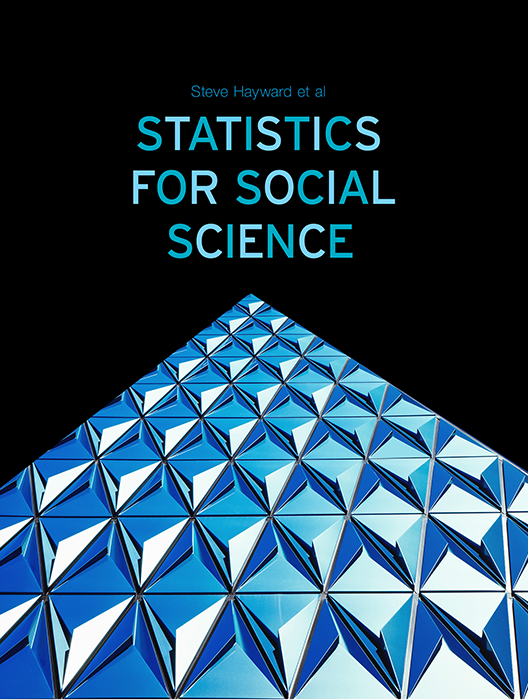# Statistics for Social Science

Lead Author(s): Stephen Hayward

Student Price: Contact us to learn more

Statistics for Social Science takes a fresh approach to the introductory class. With learning check questions, embedded videos and interactive simulations, students engage in active learning as they read. An emphasis on real-world and academic applications help ground the concepts presented. Designed for students taking an introductory statistics course in psychology, sociology or any other social science discipline.

This content has been used by 8,525 students

## What is a Top Hat Textbook?

Top Hat has reimagined the textbook – one that is designed to improve student readership through interactivity, is updated by a community of collaborating professors with the newest information, and accessed online from anywhere, at anytime.

• Top Hat Textbooks are built full of embedded videos, interactive timelines, charts, graphs, and video lessons from the authors themselves
• High-quality and affordable, at a significant fraction in cost vs traditional publisher textbooks

## Key features in this textbookOur Statistics for Social Science textbook allows students to manipulate data, visualize the effects discussed, and explore Lightboard videos that feature instructor explanations to reinforce concepts and calculations.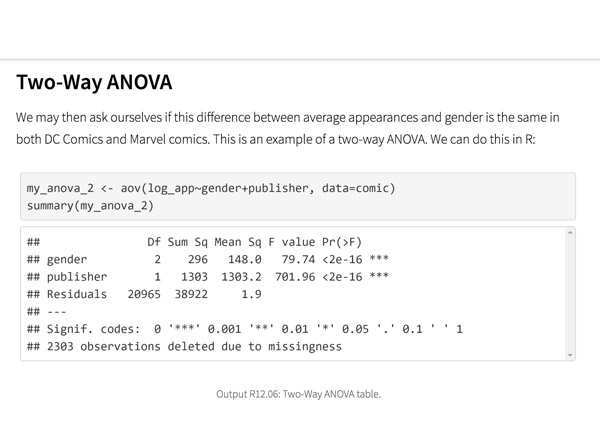Top Hat’s interactive offering includes a complementary module on using R software for data management, graphics, and conducting statistical analyses with examples and practice questions.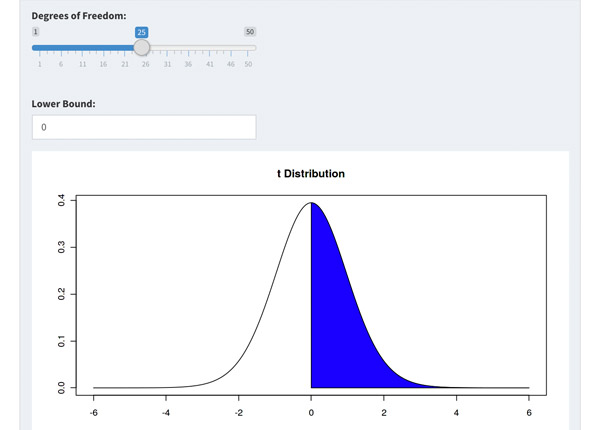Built-in assessment questions embedded throughout chapters so students can read a little, do a little, and test themselves to see what they know!

## Comparison of Social Sciences Textbooks

Consider adding Top Hat’s Statistics for Social Sciences textbook to your upcoming course. We’ve put together a textbook comparison to make it easy for you in your upcoming evaluation.

### Top Hat

Steve Hayward et al., Statistics for Social Sciences, Only one edition needed

### Pearson

Agresti, Statistical Methods for the Social Sciences, 5th Edition

### Cengage

Gravetter et al., Essentials of Statistics for The Behavioral Sciences, 9th Edition

### Sage

Gregory Privitera, Essentials Statistics for the Behavioral Sciences, 2nd Edition

### Pricing

Average price of textbook across most common format

#### Up to40-60%more affordable

Lifetime access on any device

#### $200.83 Hardcover print text only ####$239.95

Hardcover print text only

#### $92 Hardcover print text only ### Always up-to-date content, constantly revised by community of professors Content meets standard for Introduction to Anatomy & Physiology course, and is updated with the latest content ### In-Book Interactivity Includes embedded multi-media files and integrated software to enhance visual presentation of concepts directly in textbook Only available with supplementary resources at additional cost Only available with supplementary resources at additional cost Only available with supplementary resources at additional cost ### Customizable Ability to revise, adjust and adapt content to meet needs of course and instructor ### All-in-one Platform Access to additional questions, test banks, and slides available within one platform ## Pricing Average price of textbook across most common format ### Top Hat Steve Hayward et al., Statistics for Social Sciences, Only one edition needed #### Up to40-60%more affordable Lifetime access on any device ### Pearson Agresti, Statistical Methods for the Social Sciences, 5th Edition ####$200.83

Hardcover print text only

### Pearson

Gravetter et al., Essentials of Statistics for The Behavioral Sciences, 9th Edition

#### $239.95 Hardcover print text only ### Sage McConnell, Brue, Flynn, Principles of Microeconomics, 7th Edition ####$92

Hardcover print text only

## Always up-to-date content, constantly revised by community of professors

Constantly revised and updated by a community of professors with the latest content

### Top Hat

Steve Hayward et al., Statistics for Social Sciences, Only one edition needed

### Pearson

Agresti, Statistical Methods for the Social Sciences, 5th Edition

### Pearson

Gravetter et al., Essentials of Statistics for The Behavioral Sciences, 9th Edition

### Sage

Gregory Privitera, Essentials Statistics for the Behavioral Sciences, 2nd Edition

## In-book Interactivity

Includes embedded multi-media files and integrated software to enhance visual presentation of concepts directly in textbook

### Top Hat

Steve Hayward et al., Statistics for Social Sciences, Only one edition needed

### Pearson

Agresti, Statistical Methods for the Social Sciences, 5th Edition

### Pearson

Gravetter et al., Essentials of Statistics for The Behavioral Sciences, 9th Edition

### Sage

Gregory Privitera, Essentials Statistics for the Behavioral Sciences, 2nd Edition

## Customizable

Ability to revise, adjust and adapt content to meet needs of course and instructor

### Top Hat

Steve Hayward et al., Statistics for Social Sciences, Only one edition needed

### Pearson

Agresti, Statistical Methods for the Social Sciences, 5th Edition

### Pearson

Gravetter et al., Essentials of Statistics for The Behavioral Sciences, 9th Edition

### Sage

Gregory Privitera, Essentials Statistics for the Behavioral Sciences, 2nd Edition

## All-in-one Platform

Access to additional questions, test banks, and slides available within one platform

### Top Hat

Steve Hayward et al., Statistics for Social Sciences, Only one edition needed

### Pearson

Agresti, Statistical Methods for the Social Sciences, 5th Edition

### Pearson

Gravetter et al., Essentials of Statistics for The Behavioral Sciences, 9th Edition

### Sage

Gregory Privitera, Essentials Statistics for the Behavioral Sciences, 2nd Edition

## About this textbook

### Lead Authors

#### Steve HaywardRio Salado College

A lifelong learner, Steve focused on statistics and research methodology during his graduate training at the University of New Mexico. He later founded and served as CEO of Center for Performance Technology, providing instructional design and training development support to larger client organizations throughout the United States. Steve is presently lead faculty member for statistics at Rio Salado College in Tempe, Arizona.

#### Joseph F. Crivello, PhDUniversity of Connecticut

Joseph Crivello has taught Anatomy & Physiology for over 34 years, and is currently a Teaching Fellow and Premedical Advisor of the HMMI/Hemsley Summer Teaching Institute.

## Explore this textbook

Read the fully unlocked textbook below, and if you’re interested in learning more, get in touch to see how you can use this textbook in your course today.

# Confidence IntervalsHave you ever checked the weather forecast expecting a nice and sunny day, to later find out that it was pouring? How come even when the forecast says 0% chance of rain, it can still rain? This is because they usually don't post how certain these numbers are! As the duration to the forecast increases, the certainty of it decreases. If you wanted the weather for a few days from now, but the network admitted that its estimate was very uncertain, you probably wouldn't pay much attention to it. 

## Chapter Objectives

After completing this chapter, you will be able to:

• Describe the role of estimation within inferential statistics
• Distinguish between confidence levels and confidence intervals
• Calculate and interpret confidence intervals for means and proportions
• Apply abstract concepts like sampling error to specific methods like surveys and polls
• Find and interpret the margin of error and apply it to a confidence interval
• Describe the impact of changing the sample size on our ability to make estimates

## What Are Confidence Intervals and Why Do We Use Them?

By this point in your reading, it has likely dawned on you that statistics have a great deal of applicability to everyday life. Statistics involve everything from the calculation of your Grade Point Average to deciding whether you should invest in the stock market. Day to day life revolves around statistical probability. It is human nature to want to predict what might occur tomorrow, next month, next year, and so forth, as it gives us a sense of control over what happens to us.

A confidence interval (C.I.) is a type of probability statistic that specifies the range of values we use to estimate the location of an unknown population parameter such as a mean or a proportion. In other words, we don’t usually know the population mean or proportion so we estimate the likelihood of it to be within a certain range on the basis of a sample mean or proportion. The width of that range is determined by something called the margin of error (E). The margin of error accompanies a confidence level, which tells us how likely our parameter is to lie within that interval. Together, they tell us how accurate our estimate is likely to be. Because sample statistics are used to estimate the location of population parameters, confidence intervals lie within the realm of inferential statistics.

You might have encountered confidence intervals quite a bit without even realizing it. Suppose that you have been feeling fatigued and you visit your physician for a blood sample to see if you are anemic. The method to determine whether your red blood cell count is in the “normal” range involves the use of a confidence interval. Or if you have read in the newspaper about the latest Gallup poll on Americans’ attitudes toward gun control, you will likely see a statement about the margin of error that is part of a confidence interval.

Be it in medicine, political polling, or many other areas of our everyday life, confidence intervals are calculated in a variety of situations in which we need to gauge the reliability of our estimates. Some of these situations involve critical decision-making (such as whether your blood test is far enough outside of the “normal” range to warrant medical treatment), while others provide us with knowledge for its own sake. Below we will examine how social researchers use confidence intervals in different situations to estimate the probability that the parameter in which they are interested lies within a particular range of scores.

## Point Estimates and Interval Estimates

A point estimate is a single statistic (usually a sample mean or a percentage) that is used as our best estimate of a corresponding parameter, i.e., the value in a population from which you drew that estimate. For example, a sample of people who voted in the last Canadian election (such as the Canadian Elections Survey) might give us a mean age of 50.2 years, which we could then use as an estimate of the mean age of the Canadian population who voted.

Point estimates are appealingly simple. They possess a lot of apparent precision in that they give us a specific figure (in this case one that suggests youth electoral participation is very low). But it would be somewhat misleading because we cannot be certain that the true population mean is exactly 50.2 years. The point estimate’s level of precision comes at the expense of its accuracy. Accuracy does not refer to mistakes per se, but to freedom from error, or the extent to which our estimate differs from the parameter of interest’s true value (in this case the true age of voters in the population). Data that we get from samples will always possess some degree of error.

For this reason, researchers tend to calculate an interval estimate, which provides us with a range of values within which the population parameter is likely to fall. For example, between 46.2 years and 54.2 years of age. We therefore would have a less precise (but more accurate) idea of what the average age of Canadian voters will likely be, give or take a little bit. Confidence intervals, as we shall see, are a type of interval estimate.

### Examples: Point Estimates vs. Interval Estimates

Example 1: Estimate of a Mean

The amount of time that children spend in front of a screen (including activities like texting, television, and video games) has been of increasing interest to social researchers as technology moves more deeply into our daily lives. Suppose that the mean amount of daily screen time recorded for a sample of 500 children was 4.0 hours. Some children might have as little as one hour or less of screen time, while others might spend over 5.0 hours in front of one, but our best estimate for an average child (drawn from the entire population of children across the country) is precisely 4.0 hours.

We could instead estimate that the mean amount of screen time spent by children likely falls somewhere between 2.5 and 5.5 hours. While it would be less precise than saying it is exactly 4.0 hours, it is probably more accurate to use a range of values. That would be a fairly wide estimate, and it is important not to make the estimate so wide that it becomes of little utility (wherein you are pretty much guaranteed that the parameter will fall within the interval simply because it includes nearly all possible values).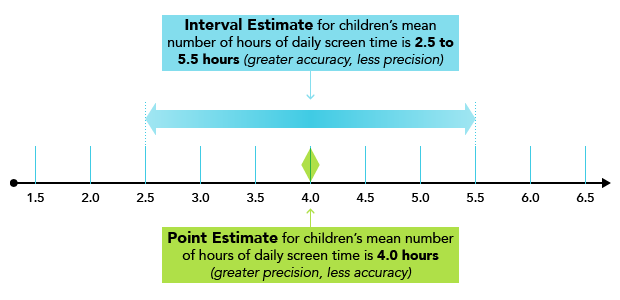Figure 8.1: Point estimate vs. interval estimate for the mean number of hours of screen time from a sample of 500 children​

Example 2: Estimate of a Proportion (in Percent)

Polling research shows that there are certain topics that are particularly likely to divide populations along political and ideological lines. One of those topics is the death penalty. Trend studies have shown that the percentage of Americans who support the death penalty has gradually decreased since the 1970s, but remains above 50%. In 2015, Gallup measured support for the death penalty for murder at 61% of all Americans (although much lower for Blacks and Hispanics). We cannot know for sure how many Americans support it, but our best estimate is 61% (expressed as a proportion would be .61).

If we were to state that “between 57% and 65% of Americans support the death penalty for murder”, we would be making an interval estimate. It is not as precise as saying “61%,” but it is likely to be more accurate in that we can have greater confidence that the true percentage is somewhere in that range.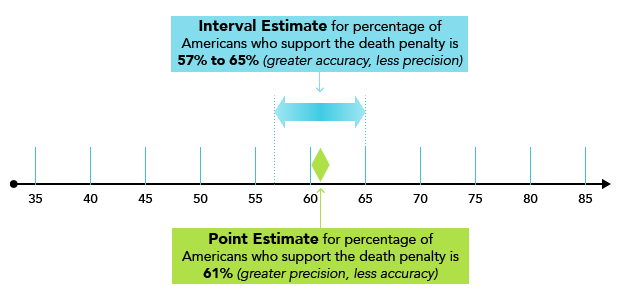Figure 8.2: Point estimate vs. interval estimate for the percentage of Americans who support the death penalty

8.01

Which of the following would be an example of a point estimate? (Select all that apply)

A

The percentage of Gallup poll respondents who said that they intended to vote in the next U.S. presidential election.

B

The percentage of all registered voters in the United States who are female is between 45 and 55 percent.

C

The mean age of a sample of registered voters from the most recent U.S. presidential election.

D

The average temperature of newborn infants is within three degrees of 96.8º F.

8.02

Imagine that you were studying the weight of children in the United Kingdom. Which of the following would be an example (or examples) of an interval estimate? (Select all that apply)

A

The mean weight of British 5-year-olds is 40 pounds.

B

The proportion of children who are overweight is estimated to be between 35% to 45%.

C

40% of all 5-year-old children in Great Britain are overweight.

D

The average weight of all British 5-year-olds is between 39.5 and 44.5 pounds.

8.03

While the main advantage of a point estimate is its _____, its main disadvantage is its _____.

A

Precision; inaccuracy

B

Accuracy; imprecision

## The Level of Confidence

The essence of sampling theory is that a well-drawn probability sample (wherein every element has a known probability of being selected, normally involving a random mechanism) will usually yield results that very closely resemble those that we would get if we measured the entire population. Researchers use samples because measuring entire populations is usually not only unfeasible but also unnecessary. The law of large numbers tells us that with a sufficiently large sample size, sample statistics (usually means) will tend to approximate population parameters very closely. You will find some values that are higher than expected, and some that are lower, but in a large sample those differences tend to average out.

However, we do take a certain amount of risk with using any sample to make inferences about populations. Because they are based on samples, interval estimates, including confidence intervals, will vary from sample to sample, as illustrated in Figure 8.3 below. The number of those lines representing the means of different samples (x̅1, x̅2, etc.) could theoretically carry on infinitely. Most of them will contain the population parameter, while a certain proportion will not. A confidence level specifies the probability that our particular sample’s interval estimate will in fact contain the population parameter.Figure 8.3: Distribution of sample means​

The researcher typically sets the desired confidence level at the outset, depending upon how much risk he or she is willing to take. The lower the confidence level, the more risk that their interval estimate does not contain the parameter. The higher the confidence level, the lower that risk. The law of large numbers tells us that the larger the sample size, the lower the risk that our sample will not contain the parameter; therefore, we can select a higher level of confidence with larger sample sizes.

## The Three Most Common Levels of Confidence

Conventionally there are three levels of confidence used in the social sciences: 90%, 95%, and 99%. Most often, academic researchers and pollsters using large samples (at least 30 cases, although opinions on that number vary) prefer the 95% or the 99% confidence level.

What this means is that in, for example, 95% of all samples drawn from the same population using the same measure (e.g. a polling question) at the same time will be equal to ± the amount of sampling error in the confidence interval in question. In the case of the 99% confidence level, 99% of all samples drawn from the same population using the same measure at the same time will also be ± the amount of sampling error in question.

In other words, how confident are we that our results are accurate? What is the chance that our intervals do not contain the true population value?

## Common Misinterpretations of Confidence Level

Many statisticians are at pains to remind non-statisticians that confidence levels are very easily misunderstood. One common misinterpretation is that your interval estimate will hold a certain percentage of all cases in the population. For example, one could mistakenly conclude that 95% of all of the weights of adult males in the United States will be between 150 and 165 pounds. A correct interpretation would be: We can be 95% confident that our interval estimate of 150 to 165 pounds will in fact contain the true population value.

A 90% confidence level does not mean for example that 90% of all sample means in a sampling distribution will fall within our confidence interval. It means that in 10% (or 1 in 10) of all samples (if we were theoretically to repeat our sample over and over, infinitely), we would expect to fail to capture the population mean within our interval estimate. Likewise, a 95% confidence level means that we would expect to fail to capture the population mean in 5% (or 5 in 100) of our samples, or 1% (1 in 100) of our samples at the 99% confidence level.

Examples: Levels of Confidence

Large-scale household surveys measuring things like income and employment status are often conducted by national statistical agencies, such as the U.S. Bureau of Labor Statistics (BLS) and Statistics Canada. Suppose that the BLS measured the mean weekly earnings before taxes for fully employed individuals to be $700. For a 95% confidence level, we could state that we are 95% confident that the true mean weekly earnings among all fully employed Americans lies between an interval of$650 and $750. In terms of a proportion (converted into a percentage), suppose that for the same survey we measured a 62% labor force participation rate (i.e. employment in the labor force, including full-time, part-time and casual). We could state that we are 99% confident that between 60% and 65% of all Americans are active in the labor force. 8.04 Level of confidence refers to the probability that our interval estimate will contain the _____. 8.05 The highest level of confidence that is conventionally used with large samples is $\_\_\_$%. 8.06 A 95% level of confidence means that 95 of every 100 samples taken would do which of the following? (Select all that apply) A Capture the sample mean in another sample. B Capture the sample proportion in another sample. C Capture the mean from the population. D Capture the proportion from the population. ## Confidence Interval vs. Confidence Level Confidence levels and confidence intervals are closely intertwined but are fundamentally distinguishable from one another. A confidence interval specifies a range of values in which we estimate that a(n) (unknown) population parameter will fall, such as a range of 57% to 65% of Americans who support the death penalty. As you learned in the previous section, a confidence level specifies the probability (usually set at 95% or 99%) that the population parameter will indeed lie within that range of 57% to 65%. It means that in 95 out of 100 samples (if we were to repeat the sampling procedure over and over, theoretically to infinity), the parameter (a mean or a proportion) would be captured within that range of values in our confidence interval. As such, it provides us with a measure of the accuracy of our interval estimate. ### Interval Width and Level of Confidence The higher the level of confidence that we select, the wider the confidence interval will be. An example in Figure 8.4 is student GPA. Here we can see that the higher the level of confidence we select, the wider the interval will be. Lower levels of confidence (e.g. 90%) will have a smaller interval because the trade-off for having a smaller and more precise range of values in which we think our parameter lies is that we can be less sure that we would capture it in subsequent samples. We can have more certainty about the parameter, but the trade-off is that it will be within a broader band of values.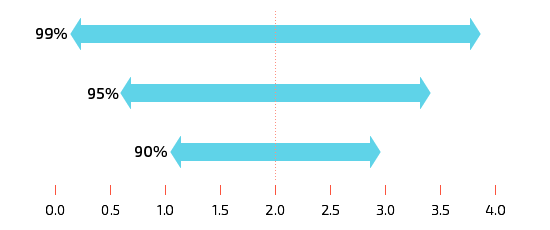Figure 8.4: Width of confidence intervals for different levels of confidence, student grade point average (any sample size)​ ### Interval Width and Sample Size The same principle would apply to sample size. Because they are based on estimates, which are subject to sampling error, the width of a confidence interval will vary from sample to sample. But by saying that we are 90%, 95%, or 99% confident in our interval, it means that most of the time (as much as 99% of the time) the widths will not vary greatly and will likely contain the population parameter. In the following figure, notice that larger samples are associated with narrower widths, while smaller samples are associated with greater widths. This will be the case regardless of confidence level; larger samples will always have the effect of narrowing your C.I. However, the width of the interval in each instance will also be affected by the level of confidence involved, so that both n and the level of confidence together determine interval widthFigure 8.5: Width of confidence intervals for different sample sizes, student grade point average (any level of confidence)​ Practice with the demonstration below to see how adjusting the C.I., sample size and standard deviation affect the interval.This Content is Locked Only a limited preview of this text is available. You'll need to sign up to Top Hat, and be a verified professor to have full access to view and teach with the content. 8.07 As our confidence level increases, the width of our confidence interval ______. A Also increases B Decreases C Stays the same 8.08 Suppose that we have collected information on how much a sample of households spend on clothing per year. If we are 90% confident that the true population mean will lie between$1,500 and $2,100, the chance that the population mean will either be less than$1,500 or above $2,100 is _____ %. 8.09 Sort the following intervals in order of width, from narrowest to widest (assuming the SD is constant in all three cases). A 99% C.I. where $n$= 100 B 95% C.I. where $n$=100 C 95% C.I. where $n$=500 ## The Margin of Error vs. Sampling Error You will recall from the Introduction to Statistics that a statistic is a numerical description of a sample. That statistic is intended to reflect an unknown value in the population, the corresponding parameter. Because it is virtually impossible to measure entire populations (e.g. asking 300 million Americans their height), most of the time we will never know for certain the true value of the parameter. Instead, we rely upon probability samples, and for that reason, we can reasonably assume that there will always be at least some gap between our sample statistics and their respective population parameters. That gap is known as sampling error. Sampling error is different from the margin of error in that the former occurs whenever a sample rather than the entire population is measured (which is the case most of the time). Each time we measure a sample, there will be some difference between the sample statistic we obtain and its corresponding population parameter. The law of large numbers tell us that if we were to take many sample measurements, that error would tend to average out and the average of the sample measurements would come closer and closer to the true population parameter. The margin of error (E) is an estimate of the amount of difference that we think is possible between our statistic and its corresponding parameter. Its relationship to the concept of sampling error, then, is that it is a statement of how much sampling error we think is possible for our given statistic. More specifically, the margin of error can be defined as the radius (in other words, half the width) of the confidence interval. As mentioned earlier, the width of the confidence interval is affected by: 1) the amount of variation in the population of interest, as well as 2) the sample size. The greater the sample size, and the smaller the standard deviation in the population, the smaller your margin of error will be. Figure 8.6 below illustrates the margins of error for different sample sizes and a confidence level of 95%, for a hypothetical example of church attendance in the United States. Suppose that all of these surveys in the figure below indicated that 50% of their respondents claimed that they attend church at least once per week. We want to know how much confidence we can have in the results, depending upon the sample size involved.Figure 8.6: Hypothetical example of the margins of error for the percentage of Americans who attend church every week, for different sample sizes​ This top part of the figure provides us with a graphic illustration of the likelihood that the true population value, the percentage of Americans who attend church weekly, lies within a certain area, taking into account the statistic of 50%. The bottom part of the figure shows 95% confidence intervals and their associated margins of error to their left, according to sample size. Because we rarely know the parameter, in this case how many Americans really do attend church weekly (assuming that they have responded to the survey honestly–that involves non-sampling error, which is another issue), we have to assume that there will be at least some sampling error. Sampling theory tells us that the larger the sample size, the less the amount of sampling error we expect there to be, and therefore the smaller its margin of error. How do we know this is really true when we cannot tell for sure how much sampling error there is for a statistic? To know that, we would need to know the population parameter. The following example involves a known parameter, and because of that, it shows us that sampling theory can indeed be trusted! Example: Margin of Error vs. Sampling Error It is relatively rare that we have a known parameter, but when we do, it provides a clear illustration of the difference between margin of error and sampling error, and in essence is a test of sampling theory. One such instance is M&M candies (the plain chocolate ones, not ones with nuts). The M&M company has made known the percentage of each of its colors that it manufactures (yes, there is a set proportion for each color!) They are as follows: 13% brown, 14% yellow, 13% red, 24% blue, 20% green, and 16% orange. Each of those percentages constitutes a population parameter, against which we can check a sample statistic if we take repeated samples of M&Ms. Check out the following video describing the color distribution and some other M&M trivia before we take some samples of M&Ms to see how much sampling error there will be. If we were to collect a series of samples of M&Ms, the resulting statistics would not only be a test of sampling theory, but they would also illustrate the key distinction between sampling error (the degree of error in a given sample) and margin of error (the half of the width of the interval within which the population parameter is likely to fall). In fact, one of our authors did just that by purchasing 40 bags of plain chocolate M&Ms, each one weighing 48 grams/1.7 ounces (about 55-59 candies per bag). She counted the percentage in each bag of each color, but for the sake of this example, let’s focus on the percentage that were brown. For each sample (individual bag), each statistic (% brown in the bag) can be subtracted from the population parameter (13%, which is what the M&M company states is the percentage of all M&Ms that are brown). The gap between the two percentages in each sample is the sampling error. Here is what she found: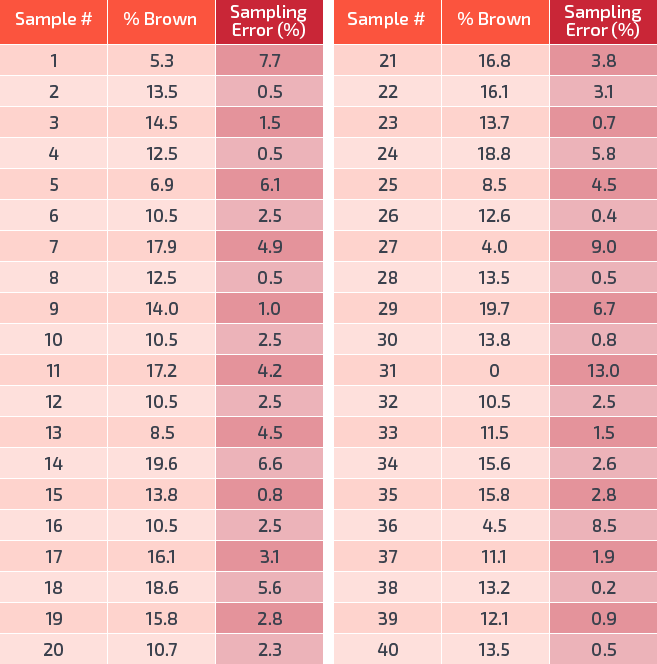Figure 8.7: Distribution of 40 samples of M&M packages (n = approx. 57 per package)​ Notice in this table that the amount of sampling error is always a positive number. The reason is that we are calculating the amount of distance between a statistic and a parameter. This distance, i.e. sampling error, varies from sample to sample. Sometimes the percentage of brown was a little bit higher than the parameter of 13% (such as the third case where 14.5% were brown, for a sampling error of 1.5%), and sometimes it was a little bit lower (such as the sixth case, which showed 10.5% brown, for a sampling error of 2.5%). Some cases were substantially higher or lower than the parameter, such as the first case, which was 7.7% below the parameter. Most of the time, however, each statistic was no more than 5% above or below the parameter. That is the essence of margin of error, in that it specifies how much sampling error that we can expect to see for that sample size. Our confidence level specifies that we will expect to see that amount of sampling error most of the time (usually set at 95% or 99%, i.e., the level of confidence). ## The Central Limit Theorem and Confidence Intervals In addition, the sampling error shown in Figure 8.7 illustrates the central limit theorem, which is the fundamental mathematical underpinning of confidence intervals. The central limit theorem dictates that with repeated samples, the sampling distribution will eventually become approximately normal and the mean of all samples will approximate the mean of the population. Example: Sampling Distribution of M&Ms A histogram of the 40 samples of M&Ms shows that the distribution was already starting to become approximately normal even with just 40 samples, with a bell shape and a mean of 12.9%, which was just slightly lower than the population mean of 13.0%.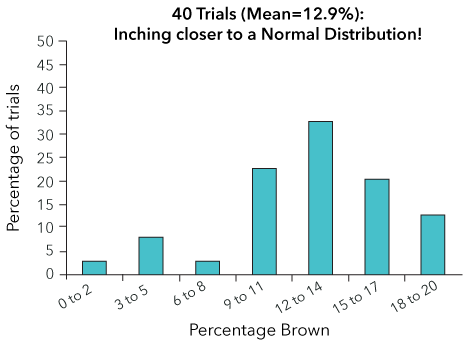Figure 8.8: Sampling distribution for 40 small samples of M&Ms​​ Recall from your readings on sampling theory that sampling distributions are ultimately theoretical distributions, meaning that they are based upon the idea of conducting repeated samples infinitely. While we cannot do that, what we can do is increase the sample size. In fact, sample size selection is based upon the concept of sampling distributions. Increasing sample size has the same effect as repeating samples over and over again—it reduces our margin of error. Example: Sampling Error as a Function of Sample Size In Figure 8.9 below, rather than counting the % of brown (as well as yellow, red, etc.) in a series of small bags, one bag (one bag = one sample) each of differing sample sizes was collected.Figure 8.9: Sample outcomes and sampling error (%, shaded) for four sample sizes of M&Ms (one sample per sample size was made)​ Notice what happens when we increase from 48g to 150g, 240g, and finally, 1,300g. As sample size increases, sampling error decreases radically. Once we reached a sample size of 1.3 kg (or 2.9 pounds), our sampling error is, for every color, within ± 5% of its corresponding population parameter. In other words, the chance that your sample will contain the population parameter increases with sample size. As sample size increases, the amount of sampling error decreases. Smaller samples, particularly the 48g sample, are less stable than larger ones, and more prone to fluctuations in that different samples will give you different results. Accordingly, we should expect that as sample size increases, the margin of error value will become smaller and the confidence interval more narrow. One might add, however, that there is a point where you have diminishing returns, and the payoff from further increases in sample size may not be worth the cost. For a survey or poll, once you reach 1,000 cases, further increases in your sample size might reduce your margin of error by less than 0.5%, and therefore 1,000 cases (or perhaps even less) are generally considered “good enough.” 8.10 Another name for sampling error is _____ error. 8.11 With repeated samples of M&M candies, eventually, the sampling distribution will become approximately _____. 8.12 What is the size of the margin of error due to? (Select all that apply) A The amount of systematic error. B The amount of variation in the sample. C The size of the sample ($n$). D The size of the statistic. ## Assumptions for a Confidence Interval As with so many other things in life, the aphorism “look before you leap” certainly applies to statistics. Numbers have a tendency to appear very scientific on paper, but they can in fact be very misleading if calculated inappropriately. As social scientists, we often talk about the socially constructed nature of the phenomena that we observe. Statistics are in many ways a social construct as well, in that they do not appear naturally, but are the product of a series of decisions made by the researcher. It is crucial that the researcher makes appropriate decisions, informed by the necessary assumptions about their data. There are two primary assumptions that must be satisfied prior to calculating confidence intervals. The first assumption is that you have used simple random sampling, or SRS. While in most cases we do not in reality use strictly SRS, as long as we are using a probability sampling method there will be the crucial element of random selection that satisfies this assumption. If not, there is greater potential for sample bias, and we cannot justifiably argue that the confidence interval is very likely to capture the true population value. The second key assumption is that we have a Normal Probability Distribution. This is crucial because of the fact that confidence intervals rely on the Central Limit Theorem in order to make an interval estimate. If the shape of the distribution is not normal, including a mean that is skewed by outliers, your interval estimate of the population parameter may be significantly distorted. As the sample size increases, however, the chances of this sort of bias occurring are greatly reduced. If anything, these assumptions are even more important given the capabilities of contemporary statistical software. A common pitfall of statistical programs is that they enhance students’ susceptibility to a “garbage in, garbage out” scenario wherein they use statistical software to unwittingly calculate meaningless statistics. The following examples illustrate the difference between situations in which the distributions satisfy the assumptions for confidence intervals, and those in which they do not. ### Examples: How Distribution Shape Affects Confidence Intervals Example 1: The Mean Weight of NHL Players One of the reasons that NHL players’ weight makes an ideal illustration of confidence intervals is that height and weight are two of the variables that most closely approximate normal distributions in the population. You will recall that in order to determine whether a variable is normally distributed, we produce a histogram from our data, as shown in Figure 8.13. This figure illustrates the distribution of the weight of a 50% sample of the NHL in 2011-12.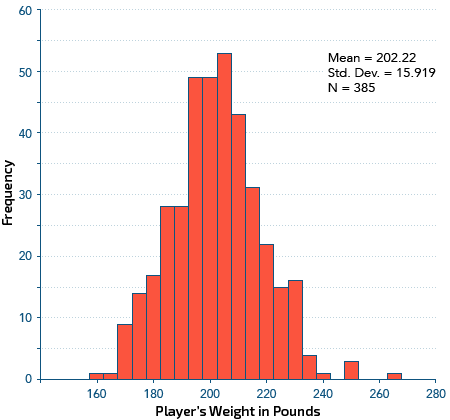Figure 8.13: Distribution of the weight of a sample of NHL players (n=385)​ Notice that the shape of the distribution is generally bell-shaped and symmetrical, with a few players on the heavier end in the extreme right side tail. In addition, we calculated the mean and median and they coincide at 202.22 and 202.00 respectively. With “real-life” data, distributions are rarely as “perfect” as ones that we create for the sake of examples in teaching. However, it comes very close in this case. It is also important to remember that the normal distribution exists primarily as an ideal, rather than something that we encounter frequently in reality. It is, in that sense, rather like buying a house. We have our mental image of the “perfect” home–its style, its yard, and so on, and we strive to find one with characteristics that meet our ideal as closely as possible. In reality, however, we are unlikely to find one that matches our ideal 100% perfectly. Example 2: The Mean Salary of NHL Players For the sake of contrast, consider what would happen if we tried to construct a confidence interval for the mean salary of NHL players. Income is generally a positively skewed variable in the population, and for those reasons, we often rely on median rather than mean values in our descriptive statistics. In Figure 8.14 we can see that players’ salaries are highly positively skewed, with the tail extending far to the right.Figure 8.14: Distribution of the salaries of a sample of NHL players (n=385)​ Skewness is also illustrated by the mean of$2,266,942 USD. The highest paid player in this sample (and in the entire NHL that season) earned $12,000,000. Removing just a few of these top-paid players would substantially reduce that mean. Therefore, among different random samples of the NHL, the mean salary might vary quite a lot depending upon who happens to be included/excluded each time, and it would in turn make the appropriateness of confidence intervals highly questionable here. However, advanced statistical software would readily allow us to do so, even though the results could be misleading. 8.13 One of the two key assumptions for confidence intervals is that the researcher has used _____ ____ ____. (Hint: 3 words) 8.14 Which one of the following sampling methods is more likely to be appropriate for calculating confidence intervals? A Use the telephone book to gather all of the phone numbers of 300 members of a small town and draw 150 of them out of a large hat. B Use a fashion magazine's website to poll the next 1,000 visitors to the site. ## Confidence Interval for the Mean To recap, confidence intervals (C.I.) are used to determine the probability that a given sample includes the population parameter, in this case, the population mean. The sample mean is used as a point estimate, providing the best single estimate of the parameter. What we are essentially doing is structuring a band of values (i.e. an interval) around the sample mean, with the width of that interval determined by the margin of error. Once that is established, we can argue that the true mean of the population lies within that interval with a given probability. The basic formula for calculating a confidence interval for the mean is: The steps involved in calculating the confidence interval are as follows: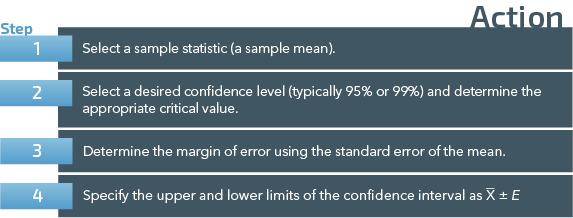Figure 8.10: Calculating confidence intervals for the mean ### Three Scenarios There are three scenarios to consider when calculating a confidence interval for a mean. The differences in procedure have to do with the method of calculating the standard error and/or the distribution used as a basis for finding the critical value that determines the cut-off points for the margin of error. The scenarios and their chief differences are summarized just below. 1. Known population standard deviation • Use z and the normal distribution to determine the critical value • Use σ to calculate the standard error 2. Large sample with ≥30 and unknown population standard deviation • Use z and the normal distribution to determine the critical value • Use s as an estimate of σ to calculate the standard error 3. Small sample with n < 30 and unknown population standard deviation • Use t and the t-distribution to determine the critical value • Use s as an estimate of σ to calculate the standard error ### Critical Values of z When using the normal distribution and z to determine cutoff points, use the critical values below to save having to look them up in a table! When using t, you’ll need to use a table (sorry!), since the lookup depends on the df involved. You can find a discussion of the t-distribution, including the method for determining the critical value of t, in Chapter Seven.Figure 8.11: Common levels of confidence and their critical values​ ### Calculating a Confidence Interval for the Mean with a Known Population Standard Deviation Sometimes (although rarely) we already know the population standard deviation, especially if the population of interest is not overly large (e.g., the entire student body of a university), or if many previous studies measuring the same variable have found consistent results (e.g., IQ). Margin of Error for Means: Known Population Standard Deviation The standard error of the mean is calculated as follows: The margin of error formula can be summarized as: The confidence interval formula for a mean with σ known can be summarized as: ### Calculating a Confidence Interval for the Mean with a Large Sample and Unknown Population Standard Deviation In the great majority of cases, the population standard deviation is not known. In that case we can substitute the sample standard deviation s for σ in determining the standard error of the mean. If the sample is large, i.e., n ≥ 30, the law of large numbers tells us the distribution of sample means will be approximately normal and we can therefore use the normal distribution to determine cut-off points for the margin of error. Margin of Error for Means: Large Sample and Unknown Population Standard Deviation The standard error of the mean is calculated as follows: The margin of error for means formula can be summarized as: The confidence interval formula for a mean with a large sample and unknown σ can be summarized as: ### Calculating a Confidence Interval for the Mean with a Small Sample and Unknown Population Standard Deviation When the sample size is small, i.e., n < 30, the distribution of sample means is no longer normal and instead follows the t-distribution with n-1 degrees of freedom. The critical value is therefore taken from that distribution, and s is again used as an estimate of σ in calculating the standard error. Margin of Error for Means: Small Sample and Unknown Population Standard Deviation The standard error of the mean is calculated as follows: The margin of error for means formula can be summarized as: The confidence interval formula for a mean with a small sample and unknown σ can be summarized as: ### Examples: Calculating Confidence Intervals for the Mean Example 1: Confidence Interval for the Mean with σ Known One population that can be quite readily measured, and that contains a known population standard deviation, is the National Hockey League (NHL). During the 2011-12 season, the NHL contained a total of 677 players. Data posted on various websites include a listing of players’ weights which, when analyzed by a computer program, had a standard deviation of 15.73 pounds. This is a relatively rare instance of a known population standard deviation (σ). Suppose that in order to conduct random drug testing, we select a random sample of 100 of the players and obtain a mean weight of 200.57 pounds. We want to ensure that our sample resembles the population in key ways, so we want to take a closer look at their mean weight. To do so, we calculate a 95% confidence interval for the mean weight of NHL hockey players. Apply the formula: C.I. = x̅ ± E Step 1: Select the sample statistic. x̅ = 200.57 pounds. Step 2: Select the desired confidence level. 95% (Z = 1.96). Step 3: Determine the margin of error. Step 4: Specify the upper and lower limits of the confidence interval. Interpretation: We can be 95% confident that the mean weight of NHL players lies between 197.49 pounds and 203.65 pounds. You can use the above statement of confidence to calculate and interpret C.I.’s in a “step-by-step” manner, which makes C.I.’s easy to use, once you get the hang of them! Example 2: Confidence Interval for the Mean with Large Sample and Unknown σ Faber University schedules 15 introductory statistics classes each semester. Dean Wormer has assigned a graduate student to provide an estimate of the average grade. Students’ grades are reported as point totals out of a possible total for the course of 1000 points. The grad student decides to report an estimate based on a 99% confidence interval. After randomly selecting 81 students from the class rosters and collecting their final course scores, which average 840 points with a standard deviation of 92.4, the student proceeds as follows: Apply the formula: C.I. = x̅ ± E Step 1: Select the sample statistic. x̅ = 840 points. Step 2: Select the desired confidence level. 99% (Z = 2.58). Step 3: Determine the margin of error. Step 4: Specify the upper and lower limits of the confidence interval. Interpretation: We can be 99% confident that the mean score of students in this semester’s statistics classes lies between 813.5 points and 866.5 points. Example 3: Confidence Interval for the Mean with Small Sample and Unknown σ A major concern among many college and university instructors is the amount of time that students spend on paid employment, knowing that it impacts their ability to study to the degree required for academic success. Suppose an instructor conducted a survey of 15 randomly selected full-time students and asked them a series of questions, including the number of paid hours that they work per week during the academic year. Here are the results for each of the 15 respondents: 0, 3, 5, 15, 35, 15, 8, 20, 40, 30, 20, 25, 21, 32, 27 The resulting mean is 19.73 hours per week, with a standard deviation of 12.10 hours. Bearing in mind that our sample size is small, these results suggest that there is quite a lot of variety in the number of hours worked by students on campus. Next, the instructor decides to conduct a 90% confidence interval for the mean hours worked per week. Apply the formula: C.I. = x̅ ± E Step 1: Select the sample statistic. x̅ = 19.73 hours Step 2: Select the desired Confidence Level. 90% Step 3: Determine the margin of error with tc (df=14) = 1.761. Step 4: Specify the upper and lower limits of the confidence interval. Interpretation: We can be 90% confident that the mean number of hours worked by students on that campus lies between 14.23 hours and 25.23 hours. 8.15 Sort these items into the order that you would use them in order to calculate the confidence interval for the mean. A Specify the upper and lower limits of the confidence interval B Select a sample statistic C Select a desired confidence level D Determine the margin of error using the standard error of the mean 8.16 One of the relatively uncommon instances in which researchers know the population standard deviation is in the case of the Intelligence Quotient or IQ test. In general, the average IQ score in large, diverse populations is 100 and the standard deviation is 15. Suppose that your sample of 300 members of your community gives you a mean IQ score of 108. Calculate a 90% confidence interval for the mean and indicate which answer set comes closest to those that would fill the blanks in the following interpretation: we can be 90% confident that the mean IQ score in this community lies between _____ and _____ . A 105.77 and 110.23 B 103.28 and 105.76 C 102.55 and 107.45 D 106.56 and 109.44 8.17 Suppose that you are a city planner who obtains a sample of 20 randomly selected members of a mid-sized town in order to determine the average amount of money that residents spend on transportation each month (such as fuel, vehicle repairs, and public transit). To 3 decimal places, what is the critical value for the 95% confidence interval? 8.18 In the same scenario as Question 8.17, suppose you obtained a mean of$167 spent on transportation and a standard deviation of $40. Calculate a 95% confidence interval for the mean and select the values that come closest to those that would fill the spaces in the following interpretation: we can be 95% confident that the mean amount of money spent on transportation lies between _____ and _____ . A$148 and $186 B$127 and $207 C$143 and $173 D$155 and $212 8.19 Click on the hotspots identifying the areas (i.e., the interval) that falls within the 99% level of confidence.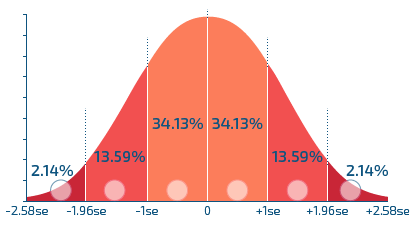8.20 Suppose that you are hired to conduct a survey of job satisfaction for a company with 500 employees across the country. One of the topics that you want to explore is telecommuting and you want to know how many miles that the employees have to travel in order to get to the office each day. Your sample of 200 of the employees showed that they travelled an average return journey of 10.5 miles daily, with a standard deviation of 6.0 miles. The margin of error for your findings at the 99% confidence level is ± _____ miles. A 0.82 B 1.09 C 3.07 D 2.21 ## Confidence Intervals for Proportions The essential logic of confidence intervals for proportions is the same as for confidence intervals for means, but this time the variables involved are nominal or ordinal, hence they are generally used when percentages are being reported. The most common place that you have likely encountered a C.I. for proportions is an opinion poll from a large sample, with a statement of margin of error usually accompanying the results. One such pollster is Bloomberg, who found in November 2015 that just 37% (or .37) of the 1,013 American adults they polled approved of their country accepting 10,000 Syrian refugees within the next year. The accompanying methodological statement to the Bloomberg poll was as follows: “These results are accurate to within ± 4 percentage points at the 95% confidence level,” or 19 times out of 20 (another commonly used wording). Such a statement involves three crucial components: a statistic (37%), a margin of error (± 4%), and a confidence level (95%), all of which are parts of confidence intervals for proportions that you will learn to calculate below. The basic formula for calculating a confidence interval for proportions is: The steps involved in calculating the confidence interval are similar to those for the mean:Figure 8.12: Calculating confidence intervals for proportions The same critical values are used for the confidence interval for proportions as were used for the confidence interval for the mean, as we are assuming a normal distribution and that the central limit theorem applies. Margin of Error for Proportions E = zc ) where zc is the critical value and σ is the standard error for proportions. The standard error for proportions is calculated as follows: where = the sample proportion, = 1 – and n = the number of cases in the sample. The margin of error for proportions formula can be summarized as: The confidence interval formula for proportions can be summarized as: ### Examples: Calculating Confidence Intervals for Proportions Example 1: A Hypothetical Election Poll (95% Confidence Level) Here is a hypothetical example from the world of politics, which is one of the most common uses of polling methodology. Angela May is once again running for Mayor of Toronto, and she has asked us to survey a random sample of 200 likely voters. She wants us to find out what percentage of the vote she can expect to receive on Election Day. Our survey results indicate that 55% of the folks in our sample of likely voters intend to vote for Ms. May. Let’s create a press release to inform the public of the poll results. Apply the formula: C.I. = ± z). Step 1: Select the sample statistic. = .55 Step 2: Set a desired confidence level. 95% (Z = 1.96). Step 3: Determine the margin of error. Step 4: Specify the upper and lower limits of the confidence interval. In our press release for this poll, we would include the following statement: “With a sample of this size, our results are accurate to within ±6.9%, 19 times out of 20.” In other words, in our C.I. we estimate that between 48.1% and 61.9% of the voters are likely to vote for Angela May. Example 2: Nielsen Television Ratings An example that you might encounter in your everyday life is Nielsen TV Ratings. Analyses of the mass media are common in the social sciences, as they are a prime agent of socialization and an enormous industry worldwide. The Nielsen Household Rating is not based upon the quality of a given program but solely on viewership. It depicts the percentage of a potential audience who are watching a particular television program (as opposed to watching other programs or doing other activities such as housework). Using set metering equipment that attaches a “black box” of sorts to their TV set, cable box, and satellite dish, information is sent nightly to Nielsen’s central computers. While techniques to measure media use have been rapidly changing to what they call “Total Audience Measurement” over the past few years due to the nature of media use (DVR, smartphones, etc.), in order to measure live TV viewing Nielsen has traditionally relied on set meters to sample about 5,000 Households (containing 13,000 people) at any one given time. People are often quite surprised that such a small sample of just a few thousand people can determine whether Dancing with the Stars, Survivor, or any of your other favorite shows is kept on the air or gets cancelled. How is it possible that such a small number of people can essentially “speak for” or represent over 300 million Americans, or 33 million Canadians, and so forth? The following clip on how Nielsen ratings work explains how the data are gathered and their consequences in terms of advertising revenue and ultimately the survival of any given program. One of the most consistently popular television programs over the past few years is Judge Judy. For example, suppose that last week her show received a Nielsen Household Rating of 7.8 (i.e. 7.8% of the market households watched it). Suppose that the sample size was 1,500 households in a Southern portion of the United States. Let’s construct a 95% confidence interval to gauge the reliability of this estimate. Apply the formula: C.I. = ± z ). Step 1: Select the sample statistic. = .078 Step 2: Set a desired confidence level. 95% (Z = 1.96). Step 3: Determine the margin of error. Step 4: Specify the upper and lower limits of the confidence interval. In our press release for this poll, we would include the following statement: “With a sample of this size, our results are accurate to within ±1.4%, 95 times out of 100 (or 19 times out of 20).” In other words, in our C.I. we estimate that between 6.4% and 9.2% of market households are watching Judge Judy. Note that this is a very small margin of error, which is a reflection of the healthy sample sizes that are typically used by Nielsen. As stated at the start of this chapter, when there are crucial decisions at stake, including millions of dollars in advertising revenue and the possible cancellation of shows, large sample sizes and a high level of confidence are used as much as possible. The following video illustrates the calculating of C.I.'s for means and proportions in more detail. 8.21 What is the name for the formula shown?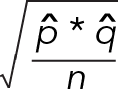8.22 The student union wants to negotiate a subsidized bus pass with your school's administration. They have polled 270 students as to whether they support this proposal, which would add a small fee to their tuition since both the college and the students would share the cost. The union found that opinions were evenly split: 50% of students were in favor of the proposal, and the rest were opposed. The margin of error for their finding at the 90% level of confidence is ± _____ %. 8.23 Referring to the data in Example 1 in the section above, what if we wanted to increase our level of confidence in the Angela May election poll to 99%? Match the following components of the C.I. with their correct values. Premise Response 1 Critical value A ± 9.0% 2 Statistic B 64.0% 3 Margin of error C 2.58 4 Upper limit D 46.0% 5 Lower limit E 0.035 6 Standard error for proportions F 0.55 8.24 Referring to Example 2 in the section above, suppose that a smaller sample size was used to conduct Nielsen ratings. What would the margin of error be (assume it is a ± value, in %) for a 95% confidence interval with a sample size of just 200 cases? ## Effect of Changing the Sample Size Many samples used by social scientists are quite large, particularly in the case of survey research. A sample that is larger than 30 cases can be considered large enough to warrant using the normal distribution and z-scores in calculating your confidence interval (as you would with a known standard deviation), but many people would argue that anything under several hundred is fairly small. What we do know from the law of large numbers is that the larger the sample, the less the amount of sampling error there is likely to be. You also know from the central limit theorem that your sample will become approximately normal with a large enough sample size. In turn, the larger the sample size, the lower the margin of error and the higher the confidence level that you can safely choose. ### Examples: The Effect of Changing the Sample Size Example 1. The Weight of Players in the National Hockey League (C.I. for the Mean) Returning to the NHL players’ mean weight, consider the possibility that we drew a larger random sample of just under three-fifths of the population of NHL players (400 players) instead of only 100 as we did in the earlier example. This new and larger sample found a mean of 202.03 pounds with the same known population standard deviation of 15.73 pounds. If we then calculate a 95% confidence interval, we will arrive at the following: Apply the formula: C.I. = x̅ ± E Step 1: Select the sample statistic. x̅ = 202.03 pounds. Step 2: Select the desired confidence level. 95%. Step 3: Determine the margin of error. Step 4: Specify the upper and lower limits of the confidence interval. You can see that by increasing our sample size from n = 100 to n = 400, we have reduced the margin of error from 3.08 to 1.54. Increasing our sample size therefore narrows our confidence interval and in those situations we can make a more precise estimate of the population mean. If we use our new sample and select the 99% confidence interval instead, our margin of error broadens again somewhat: There is always a trade-off between level of confidence and margin of error. As illustrated in the summary table below, the more that we increase our level of confidence (from 95% to 99% in this case), the more that we broaden the interval and increase our margin of error. However, if we are able to increase our sample size, our ability to use a higher confidence level increases, and our margin of error decreases, relative to smaller sample sizes. A larger n will result in a smaller margin of error than with a smaller n, regardless of confidence level.Figure 8.15: Effect of changing the sample size, for different levels of confidence​​ Looking at the lower and upper limits points out the combined impact of sample size as well as confidence level. The range of values in our interval, comprising its width, is as high as 8.1 pounds (for n =100 at 99% confidence), and as low as 3.1 pounds (for n = 400 at 95% confidence). Example 2: A Hypothetical Election Poll (C.I. for Proportions) Another example is the hypothetical election poll described earlier. For Ms. May’s purposes, the confidence intervals that you already calculated were so wide that she cannot take much comfort in the poll. She might capture quite a lot of the vote, or it might be considerably less. She really is left unsure by our results. For more precise estimates (at the same levels of confidence), May would have to request a larger sample of 750 voters this time. We can make the following calculations for the 95% level of confidence and n = 750: Apply the formula: C.I. = ± z). Step 1: Select the sample statistic. = .55 Step 2: Set a desired confidence level. 95%. Step 3: Determine the margin of error. Step 4: Specify the upper and lower limits of the confidence interval. In our press release for this poll, we would include the following statement: “With a sample of this size, our results are accurate to within ±3.5%, 95 times out of 100 (or 19 times out of 20).” In other words, in our C.I. we estimate that between 51.5% and 58.5% of the voters are likely to vote for Angela May. If we decide that we want a higher level of confidence, we could select 99%, in which case our confidence interval would broaden. We can make the following calculations for the 99% level of confidence and = 750: If we use our new sample and select the 99% confidence interval instead, our margin of error broadens again somewhat: Our press release for our poll will state the following margin of error: “With a sample of this size, our results are accurate to within ±4.6, 99 times out of 100.” In other words, we estimate that between 50.4% and 59.6% of the voters are likely to vote for Angela May.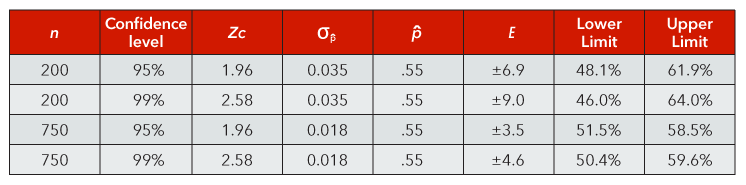Figure 8.16: Comparison of confidence intervals for samples of different sizes​ 8.25 Imagine that you are conducting a poll to determine the percentage of adults who gamble at least once a month. As your sample size increases (let us say from 100 to 400 cases), which of the following becomes true? A Confidence interval becomes wider B Margin of error becomes smaller C Amount of sampling error increases D Margin of error increases 8.26 Sort the following four samples in terms of the size of their margin of error, from smallest to largest. A 90% confidence level; $n$= 200 B 95% confidence level; $n$=200 C 95% confidence level; $n$=500 D 90% confidence level; $n$=500 8.27 Suppose that you run a survey research firm and have a reasonably good budget but you want to impress your client with your cost efficiency as well as data quality. You can select from three possible options. Which option would you select? A $n$= 5000, margin of error = ± 0.5 B $n$= 1,500, margin of error = ± 1.1 C $n$= 500, margin of error = ± 6.0 8.29 The reason that you would select the option that you did above is due to what is known as _____. ## Case Study: The Religious Landscape Study In 1959, sociologist C. Wright Mills (influenced by 19th century social theorists such as Karl Marx) declared that due to the forces of modernization, including the rise of scientific thought and the separation of church and state, “the sacred shall disappear altogether except, possibly, in the private realm” (Mills, 1959, pp. 32-33). In other words, he thought that organized religion would decline and eventually disappear. This notion has come to be known as the “secularization thesis”, and it has been a prominent debate within the sociological study of religion. Considerable cross-national evidence has indeed supported the argument that the more industrialized a society becomes, the less religious its members tend to be. One of the critiques that have been made about the secularization thesis, however, is that the United States is a striking exception to this pattern. In 2014, the Pew Research Center, a non-partisan think tank that studies a broad array of social issues, conducted The Religious Landscape Study. The study was based on a large-scale survey of over 35,000 Americans. Pew gathered an unusually large sample so that they could get a highly precise estimate of the different types of religious belief in the country. Their large sample size was also necessary in order to describe the demographic characteristics of the various different religious groups to which people belong, some of which would be too small to be measured in a small-scale survey. The following video provides a brief overview of the study’s main findings: As shown in the video, by some measures the American public is indeed becoming less religious and more secular, including a decline in the percentage of people who believe in God, regularly pray, and attend church. However, that decline is largely accounted for by an increase in the percentage of Americans who are not affiliated with an organized religion or faith, rather than a decline in those activities among those who are religiously affiliated. The percentage of respondents who reported being religiously affiliated was 77% in 2014, compared with 83% in 2007. This finding does not necessarily mean that all of the remaining 23% are atheists, but it suggests there is a substantial minority who are not strongly or actively religious. Given the large sample size, we can select the highest confidence level for our interval estimate. Apply the formula: C.I. = ± z). Step 1: Select the sample statistic. = .77 Step 2: Set a desired confidence level. 99%. Step 3: Determine the margin of error. Step 4: Specify the upper and lower limits of the confidence interval. Therefore, 76.4% < P < 77.6%. In a press release for this survey, we could include the following statement: “With a sample of this size, our results are accurate to within ±0.06%, 99 times out of 100.” In other words, in our C.I. we estimate that between 76.4% and 77.6% of Americans are affiliated with a religious organization. Also note in the video clip that among the 23% of the sample who were not affiliated with a religion, 57% felt that religion was “not important” in their lives. The sample size for that group was 7,556 respondents. If you calculate the 99% confidence interval for that statistic, you will get a margin of error of ±1.5%. You can in fact find this margin of error, along with ones for other population subgroups, in the Appendix of Pew’s report here. ### Case Study Question 8.01 Case Study Question 8.01 What major factor coincides with the falloff of traditional religious beliefs in America? Click here to see the answer to Case Study Question 8.01. ### Case Study Question 8.02 Case Study Question 8.02 What influences the statistical decline of surveyed respondents’ religious beliefs and practices, as well as their religious composition? Click here to see the answer to Case Study Question 8.02. ### Case Study Question 8.03 Case Study Question 8.03 According to the article, how do most Americans view the role of religious institutions within their communities? Click here to see the answer to Case Study Question 8.03. ### References Pew Research Centre. (2015, Nov 3). U.S. Public Becoming Less Religious. Retrieved from http://www.pewforum.org/2015/11/03/u-s-public-becoming-less-religious/ ## Pre-Class Discussion Questions ### Class Discussion 8.01 Class Discussion 8.01 Why don’t we normally know the population parameter? Click here to see the answer to Class Discussion 8.01. ### Class Discussion 8.02 Class Discussion 8.02 In what sorts of situations would we use the z-distribution in calculating a confidence interval? Click here to see the answer to Class Discussion 8.02. ### Class Discussion 8.03 Class Discussion 8.03 You and a friend are in a gambling mood and you make a bet for$20. You pretend that you are going to do a poll asking 500 people on your campus whether or not they plan to buy a ticket for the next Powerball lottery. You bet that the margin of error would be bigger for the 95% confidence level than the 99% confidence level, while your friend bets the opposite. Which one of you would win the bet?

Click here to see the answer to Class Discussion 8.03.

### Class Discussion 8.04

Class Discussion 8.04

When should we not calculate a confidence interval?

Click here to see the answer to Class Discussion 8.04.This Content is Locked
Only a limited preview of this text is available. You'll need to sign up to Top Hat, and be a verified professor to have full access to view and teach with the content.

## Answers to Case Study Questions

### Answer to Case Study Question 8.01

The religious composition of the public population coincides with the declining prevalence of traditional religious beliefs and practices.

Click here to return to Case Study Question 8.01.

### Answer to Case Study Question 8.02

Age. As older generations or cohorts of adults pass away, younger adults with far less levels of attachment to a religious affiliation and its practices replace them.

Click here to return to Case Study Question 8.02.

### Answer to Case Study Question 8.03

According to the 2014 U.S. Religious Landscape Study, with a nationally representative sample of 35,071, nine-in-ten adults claim religious institutions strengthen community bonds, and three quarters claim that these institutions protect and strengthen morality in overall society.

Click here to return to Case Study Question 8.03

## Answers to Pre-Class Discussion Questions

### Answer to Class Discussion 8.01

The population is usually too large to measure.

Click here to return to Class Discussion 8.01.

### Answer to Class Discussion 8.02

When you have a large sample or a known population standard deviation. It is rare to have a known population standard deviation but a common scenario is IQ, since it has been measured in so many studies. Sampling theory shows us that larger samples have less sampling error and we can safely assume that the sample standard deviation approximates the population standard deviation.

Click here to return to Class Discussion 8.02.

### Answer to Class Discussion 8.03

Your friend would win the bet. The trade-off for choosing a higher confidence level is that there is a wider MOE.

Click here to return to Class Discussion 8.03.

### Answer to Class Discussion 8.04

When samples are non-probability and when the distribution is not normal.

Click here to return to Class Discussion 8.04.

### Image Credits

 Image courtesy of Tony Hisgett under CC BY 2.0